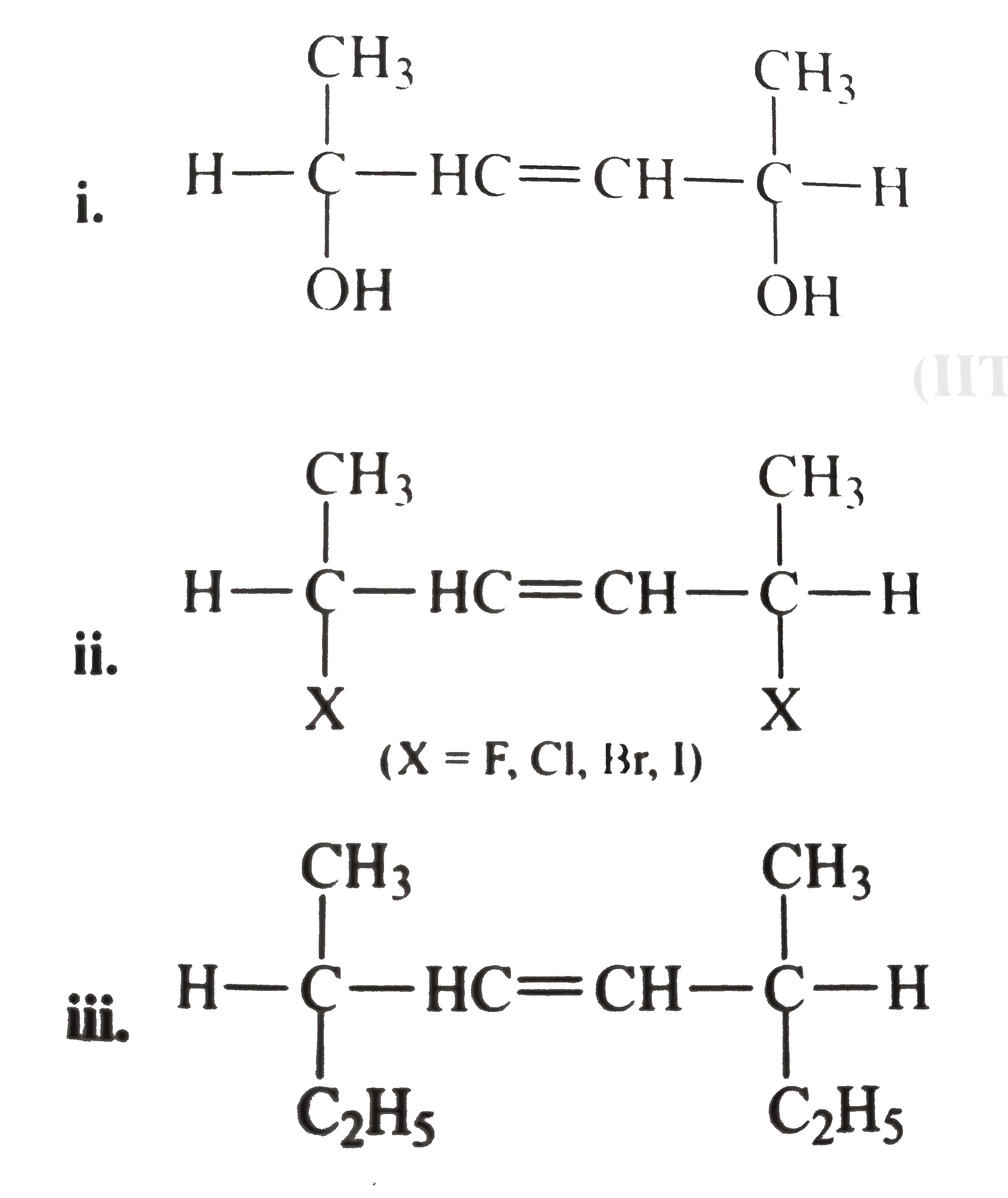# Consider the compound (A) given below: a. Give the total number of stereoisomers possible for (A). b. If the stereochemistry about the double bond in

98 views

closed
Consider the compound (A) given below: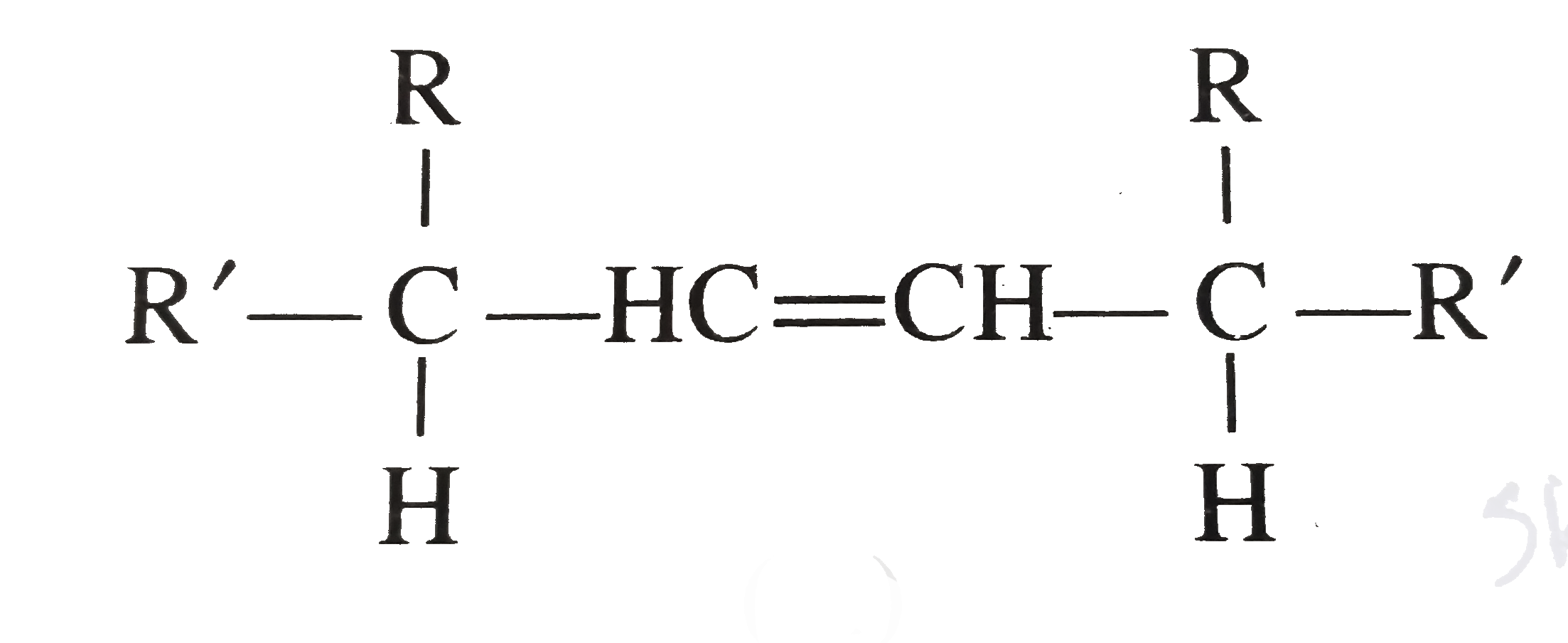a. Give the total number of stereoisomers possible for (A).
b. If the stereochemistry about the double bond in (A) is cis, give the number of enantiomers possible for (A).
c. If the stereochemistry about the double bond (A) is trans, give the number of enantiomers possible for (A).
d. Give the total number of diastereomers possible for (A).
e. Give some examples of the types of (A).

by (65.9k points)
selected

a. The compound (A) contains two asymmetric C atoms with the same terminal group.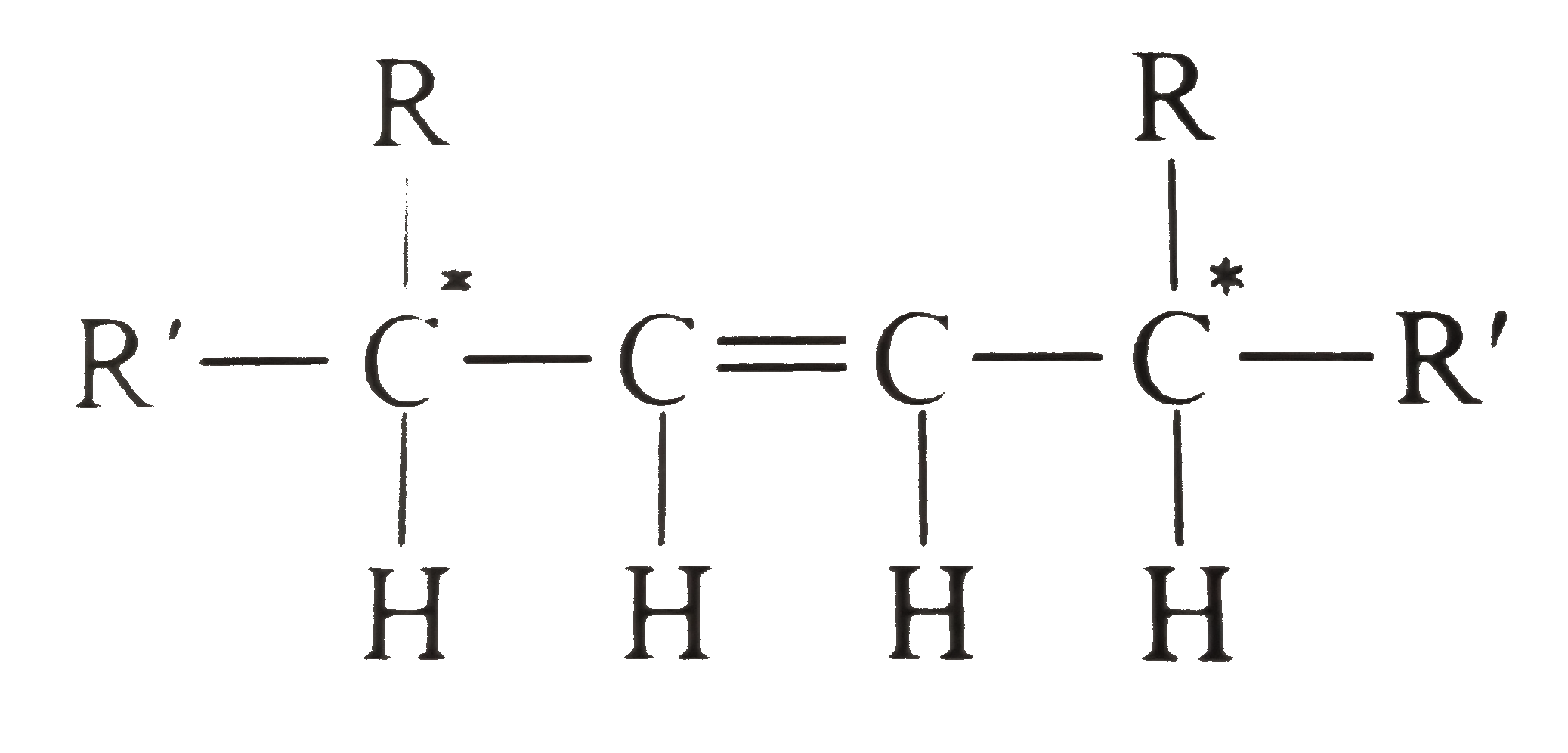Number of optically active isomers (O.I.A)= 2^(n-1)=2^(2-1)=2
(where n is the number of asymmetric C atoms).
Number of meso from=2^((n-2)//2)=2^(0)-1
Total optical isomers =2+1=3
Due to one double bond, the number of geometrical isomers =2
The number of stereoisomers =3xx2=6
b. If the stereochemistry about (C=C) bond is cis, then two pairs of diastereomers (II) and (III) are possible.
(II) is meso (O.I.A) due ot the presence of plane of symmerty . (III) is optically active (O.A) and two enantiomers are possible. Therefore, in total there are three diastereomers.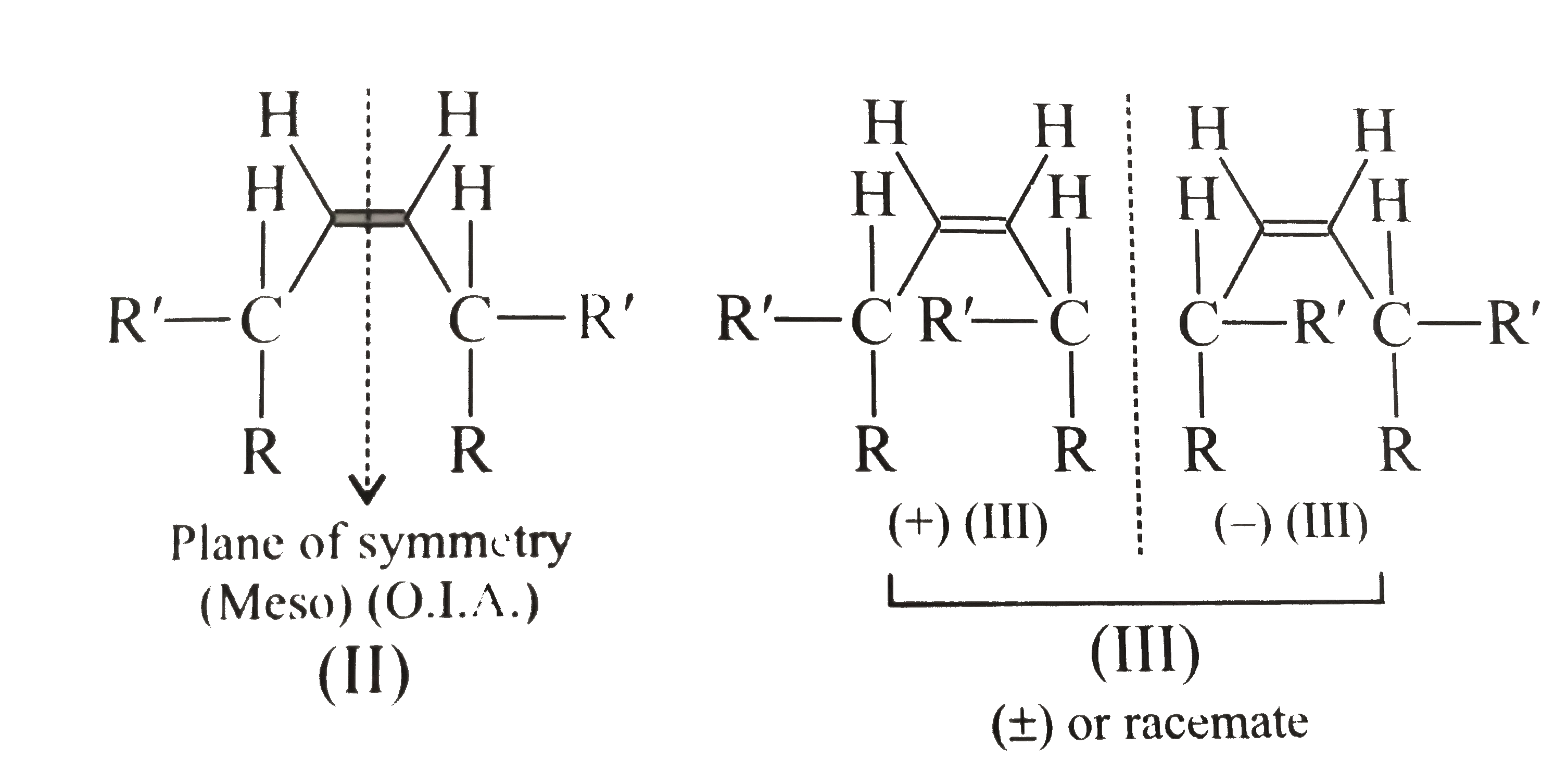II and (+) III, II and (-)III are diastereomers, wheras (+)III and (-)III are enantiomers.
c. If the stereochemistry about (C=C) bond is trans, two pairs of diastereomers (IV) and (V) are possible. (IV) is meso (O.I.A), due to the presence of centre of symmerty. (V) is O.A and two enantiomers are possible. Therefore, the in total there are three diastereomers.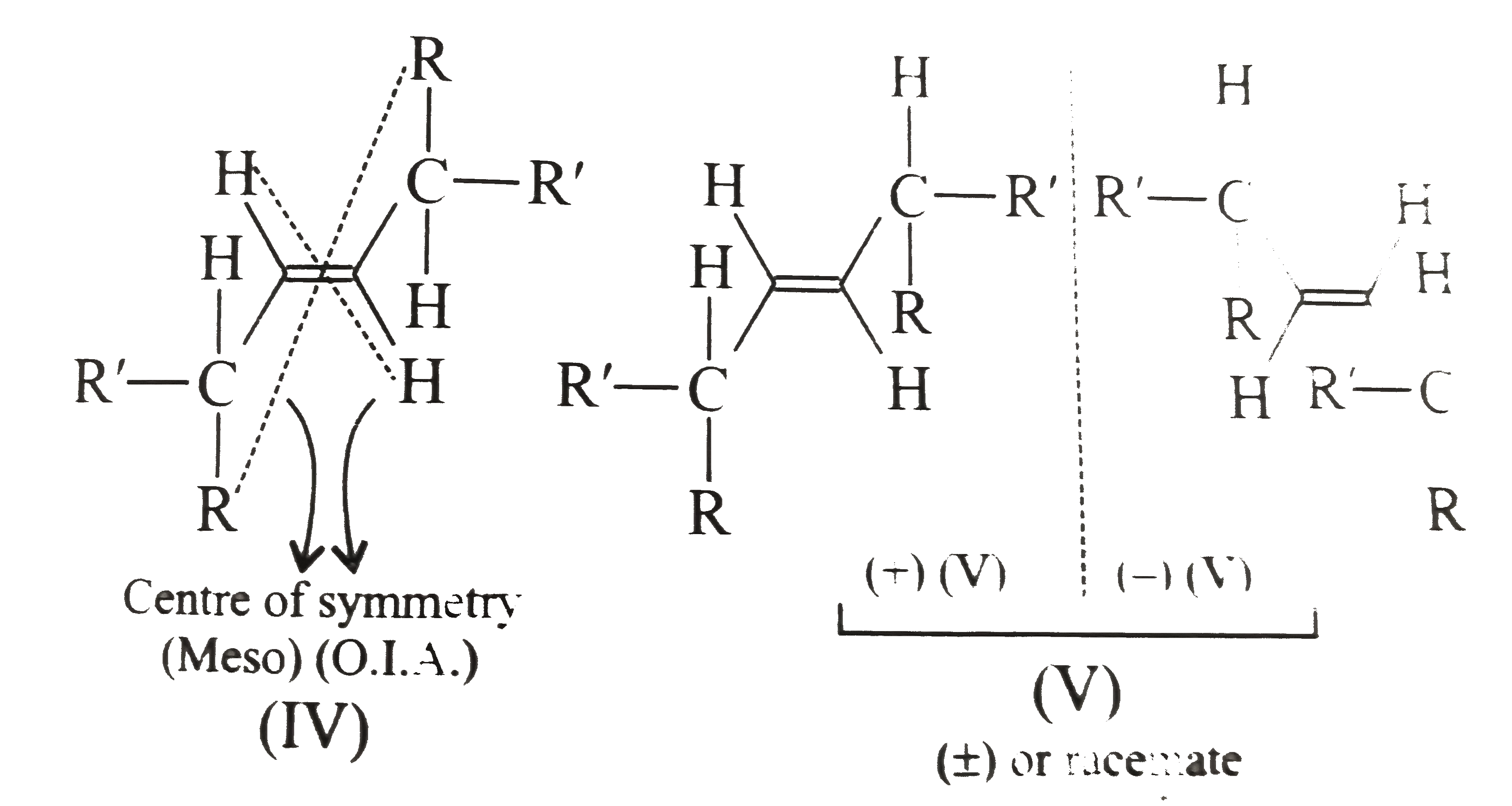[IV and (+)V] and (-)V] are diastereomers, whereas (+V) and (-V) are enantiomers.
d. Therefore, the total number of diastereomers from (b) and (c ) is six.
e. Examples: